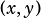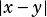Ifis a solution to the system of equations above, what is the greatest possible value of?

A)
B)
C)
D)Becky says:

Can you do this showing a math solution?Mike McClenathan says:

Sure. First, solve forby substituting:Now that you knowcan equal 3 or -2, find the corresponding-values by plugging back into the simpler equation:So the two possible ordered pairs are (3, 4) and (-2, -6). The values of, then, are: